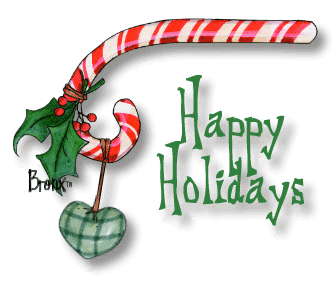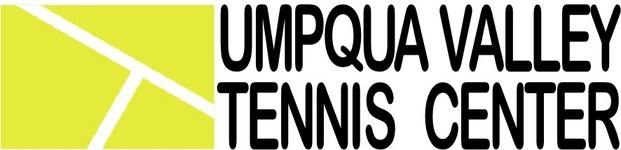## December 2018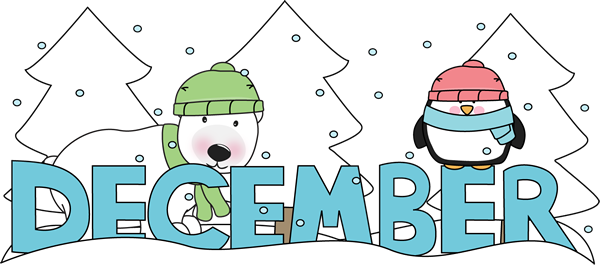table div table+table+table+table div table{width:100%;padding:0}table div table+table+table+table div table img{width:96.23%;padding:0;float:none}table div table+table+table+table div table td{width:100%;padding:0 1.88% 18px}/* styles */# Wishing you all a very Happy Holiday Season!

 table div table+table+table+table+table+table div table{width:100%;padding:0}table div table+table+table+table+table+table div table img{width:96.23%;padding:0;float:none}table div table+table+table+table+table+table div table td{width:100%;padding:0 1.88% 18px}/* styles */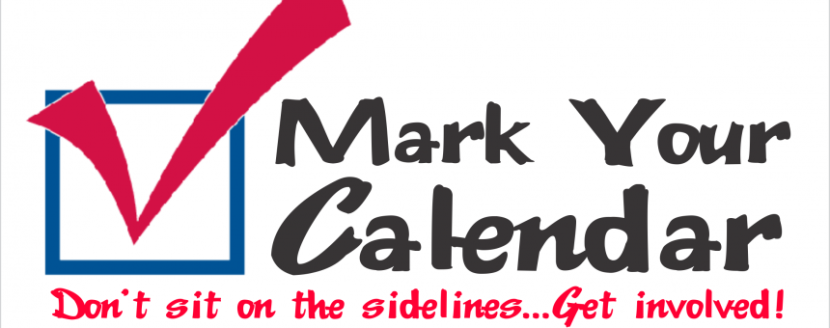/* styles */ December 24-25: Christmas Holiday - Office Closed December 31: New Years Eve - Office Closed January 1: New Years Day - Office Closed
 table div table+table+table+table+table+table+table+table+table div table{width:100%;padding:0}table div table+table+table+table+table+table+table+table+table div table img{width:96.23%;padding:0;float:none}table div table+table+table+table+table+table+table+table+table div table td{width:100%;padding:0 1.88% 18px}/* styles */## Jonah Samuel Root was born November 18th weighing 6 lbs 8 oz and is 20 inches long.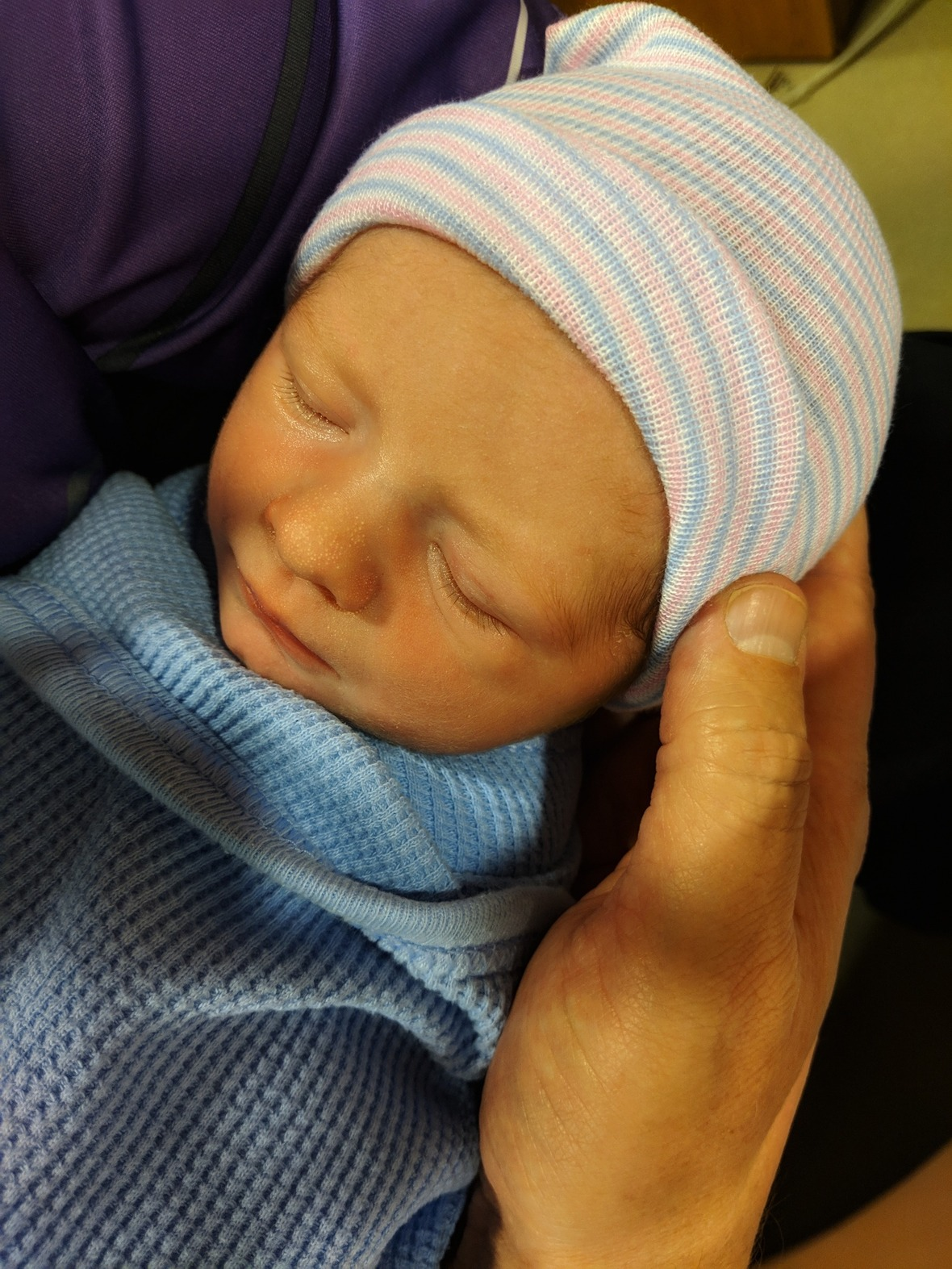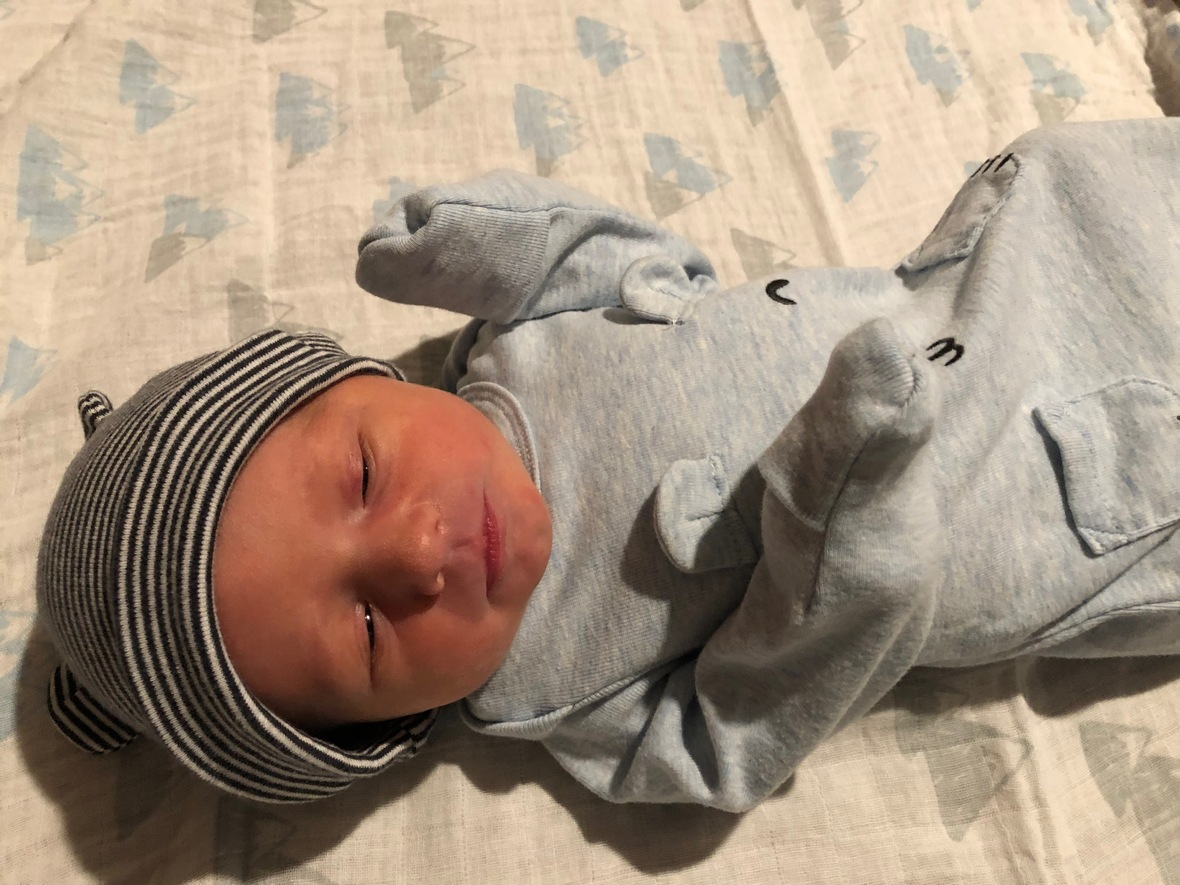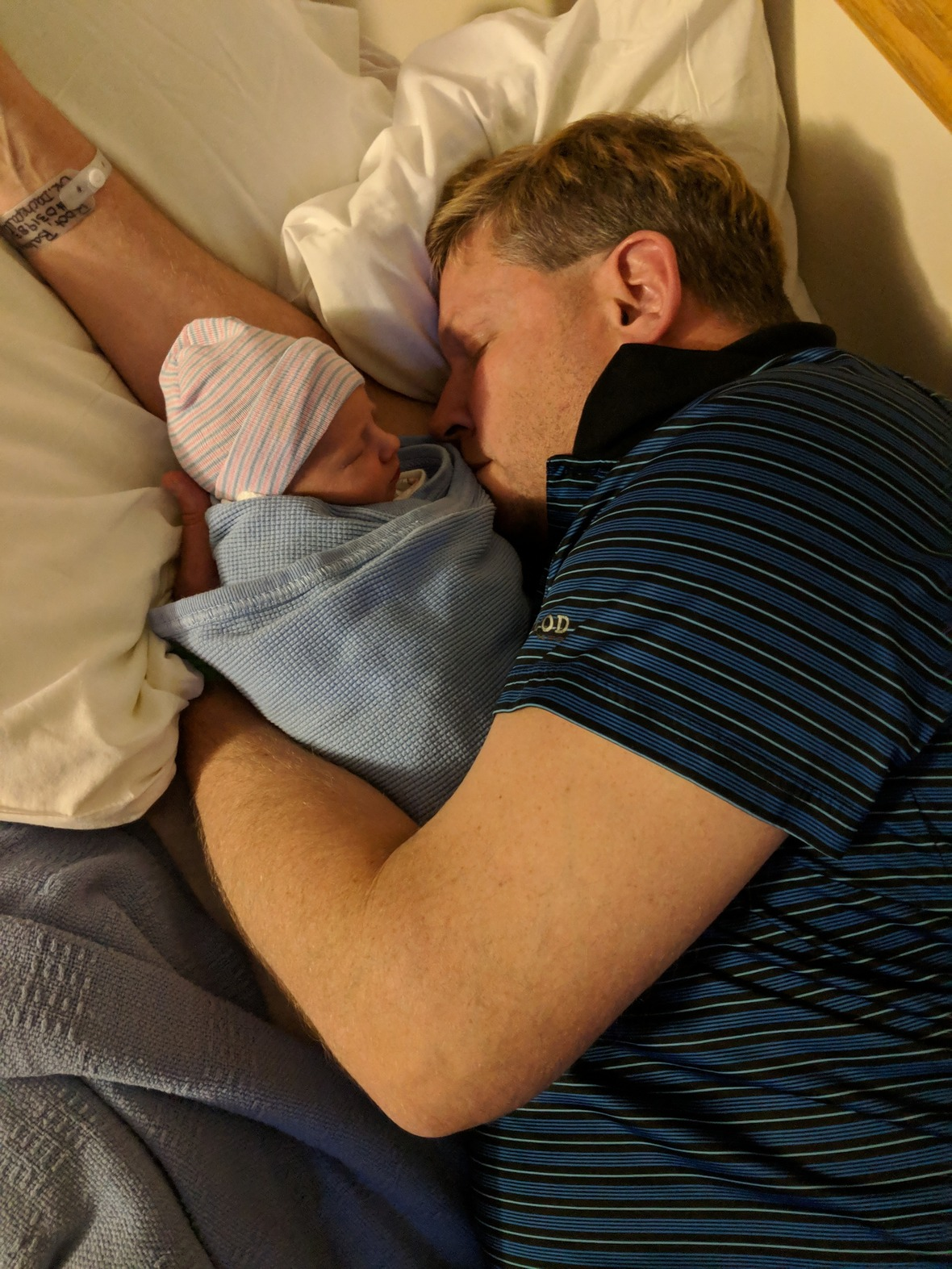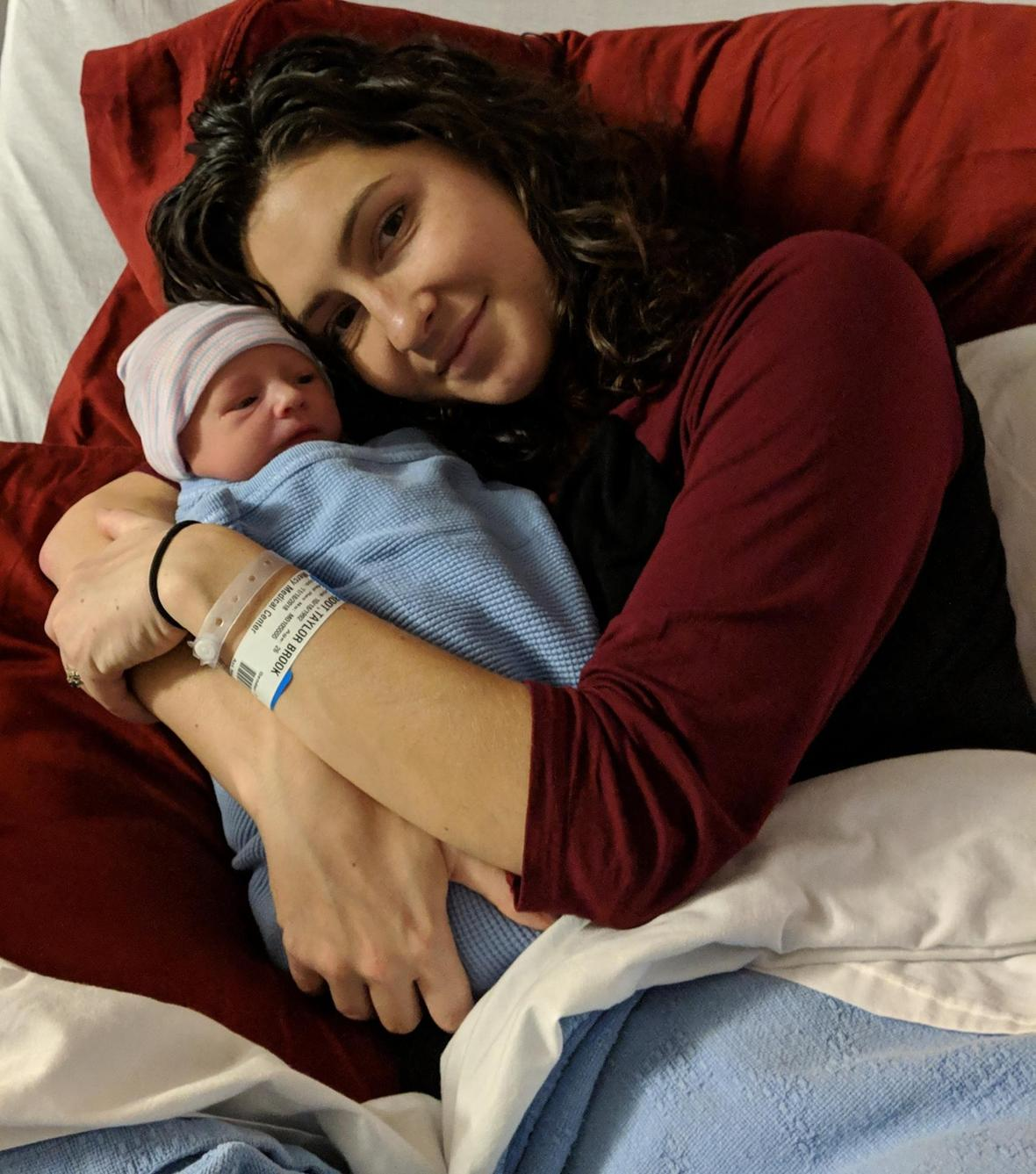table div table+table+table+table+table+table+table+table+table+table+table+table+table div table{width:100%;padding:0}table div table+table+table+table+table+table+table+table+table+table+table+table+table div table img{width:96.23%;padding:0;float:none}table div table+table+table+table+table+table+table+table+table+table+table+table+table div table td{width:100%;padding:0 1.88% 18px}/* styles */## Interested In Yoga at UVTC?

 /* styles */ We are going to try yoga at UVTC starting in January. The possible days and times will be... Mondays 6:00 - 6:45 pm and/or 7:00 - 7:45 pm or Wednesdays 6:00 - 6:45 pm and/or 7:00 - 7:45 pm The instructor will be offering Yoga and possibly Aerial Yoga depending on the interest we have. If you are interested, please reply to this email and let us know your day/time preference and if you are interested also in Aerial Yoga.
 table div table+table+table+table+table+table+table+table+table+table+table+table+table+table+table+table div table{width:100%;padding:0}table div table+table+table+table+table+table+table+table+table+table+table+table+table+table+table+table div table img{width:96.23%;padding:0;float:none}table div table+table+table+table+table+table+table+table+table+table+table+table+table+table+table+table div table td{width:100%;padding:0 1.88% 18px}/* styles */## Seven Feathers Umpqua Valley Pickleball Championships - Feb 8-9, 2019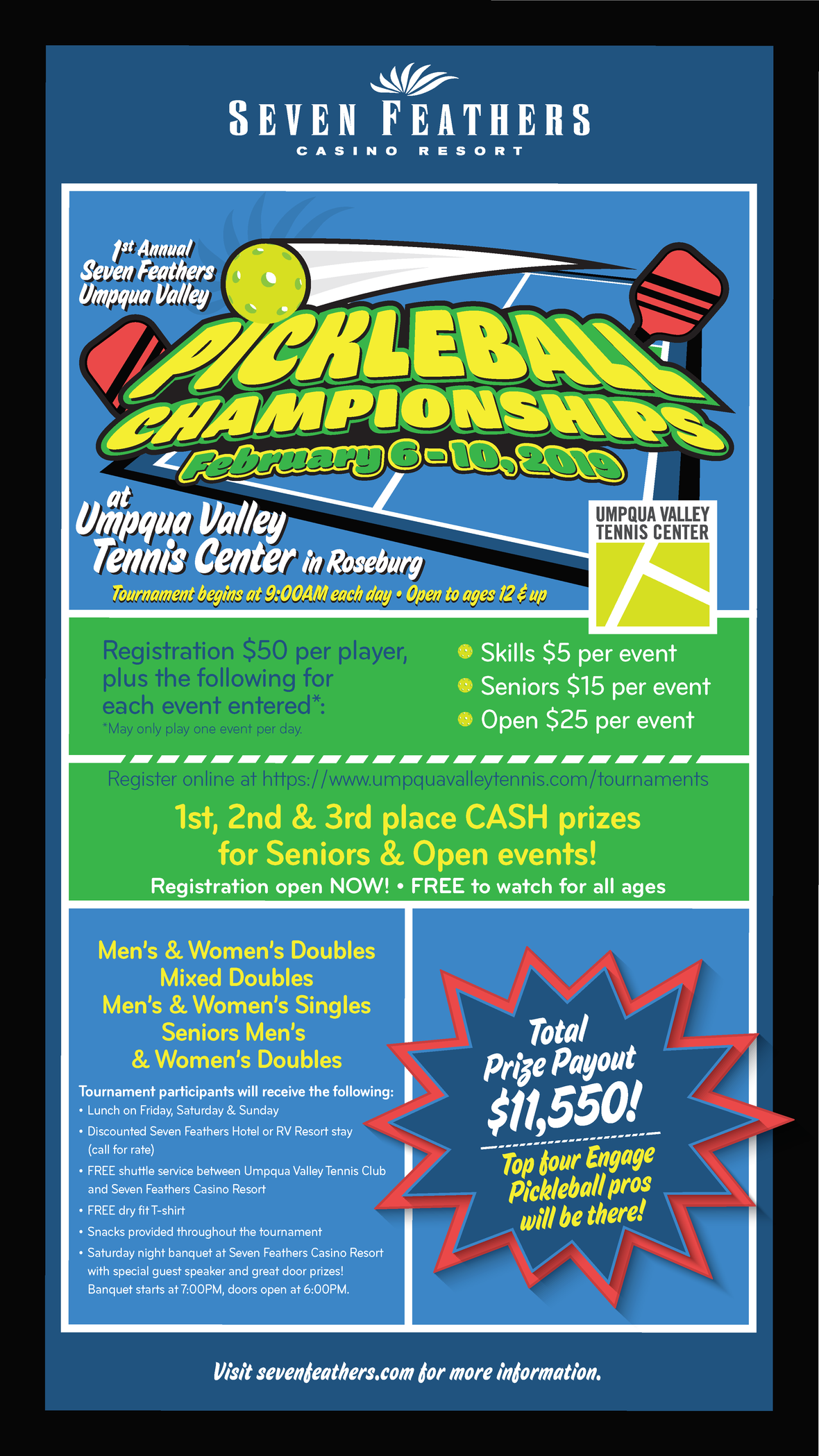table div table+table+table+table+table+table+table+table+table+table+table+table+table+table+table+table+table+table+table+table div table{width:100%;padding:0}table div table+table+table+table+table+table+table+table+table+table+table+table+table+table+table+table+table+table+table+table div table img{width:96.23%;padding:0;float:none}table div table+table+table+table+table+table+table+table+table+table+table+table+table+table+table+table+table+table+table+table div table td{width:100%;padding:0 1.88% 18px}/* styles */table div table+table+table+table+table+table+table+table+table+table+table+table+table+table+table+table+table+table+table+table+table+table+table div table{width:100%;padding:0}table div table+table+table+table+table+table+table+table+table+table+table+table+table+table+table+table+table+table+table+table+table+table+table div table img{width:96.23%;padding:0;float:none}table div table+table+table+table+table+table+table+table+table+table+table+table+table+table+table+table+table+table+table+table+table+table+table div table td{width:100%;padding:0 1.88% 18px}/* styles */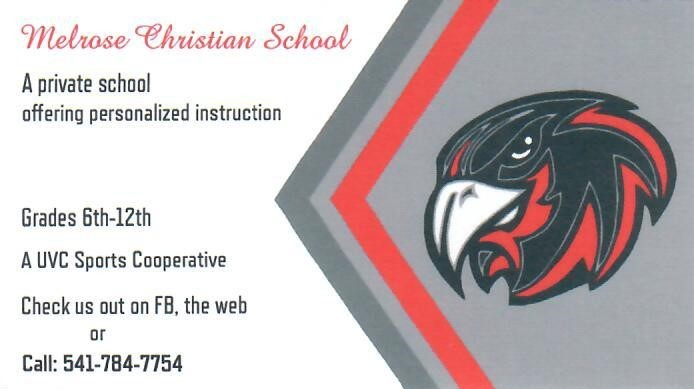table div table+table+table+table+table+table+table+table+table+table+table+table+table+table+table+table+table+table+table+table+table+table+table+table+table div table{width:100%;padding:0}table div table+table+table+table+table+table+table+table+table+table+table+table+table+table+table+table+table+table+table+table+table+table+table+table+table div table img{width:96.23%;padding:0;float:none}table div table+table+table+table+table+table+table+table+table+table+table+table+table+table+table+table+table+table+table+table+table+table+table+table+table div table td{width:100%;padding:0 1.88% 18px}/* styles *//* styles */ If you would like to place an add in the UVTC newsletter please email Ronda at Ronda@UmpquaValleyTennis.com.
 table div table+table+table+table+table+table+table+table+table+table+table+table+table+table+table+table+table+table+table+table+table+table+table+table+table+table+table div table{width:100%;padding:0}table div table+table+table+table+table+table+table+table+table+table+table+table+table+table+table+table+table+table+table+table+table+table+table+table+table+table+table div table img{width:96.23%;padding:0;float:none}table div table+table+table+table+table+table+table+table+table+table+table+table+table+table+table+table+table+table+table+table+table+table+table+table+table+table+table div table td{width:100%;padding:0 1.88% 18px}/* styles */## Guests

Please remember that when you have a guest playing with you, please let the office know. Guests also need to sign a liability waiver to have on file, they can do this at the front desk or by filling out the guest form located in the hallway and placed in the drop box.

## List all Players on your Court Reservation

Please remember to list all players playing with you on your court reservation. If they are not in the system, that means they are guests and the office needs to know so they can be added and charged the correct fee. If the guest does not pay, the guest fee falls to the responsibility of the member inviting the non-member.

## Payments for Classes & Clinics

When leaving payment for a class or clinic please do not leave cash at the front desk (if no one is there) or in the drop box without putting what it was for and who it was for. There is a drop box in the hallway with envelopes provided for your convenience.

## Garage Doors

With the weather getting nicer everyday, the 2 garage doors are being opened more often. If you are the last one out in the evening and the doors are open, please make sure to close and lock them.

## Balls left on court

Please remember to pick all tennis balls off courts (inside or outside) when you are done playing; yes, even those behind the curtains. If you would like to recycle them, there is a receptacle near the front door.

## Ball Machine

After using the ball machine, please remember to clean up any ball fuzz left on the court, put away the ball machine and pick up all the balls.

 table div table+table+table+table+table+table+table+table+table+table+table+table+table+table+table+table+table+table+table+table+table+table+table+table+table+table+table+table+table+table div table{width:100%;padding:0}table div table+table+table+table+table+table+table+table+table+table+table+table+table+table+table+table+table+table+table+table+table+table+table+table+table+table+table+table+table+table div table img{width:96.23%;padding:0;float:none}table div table+table+table+table+table+table+table+table+table+table+table+table+table+table+table+table+table+table+table+table+table+table+table+table+table+table+table+table+table+table div table td{width:100%;padding:0 1.88% 18px}/* styles */